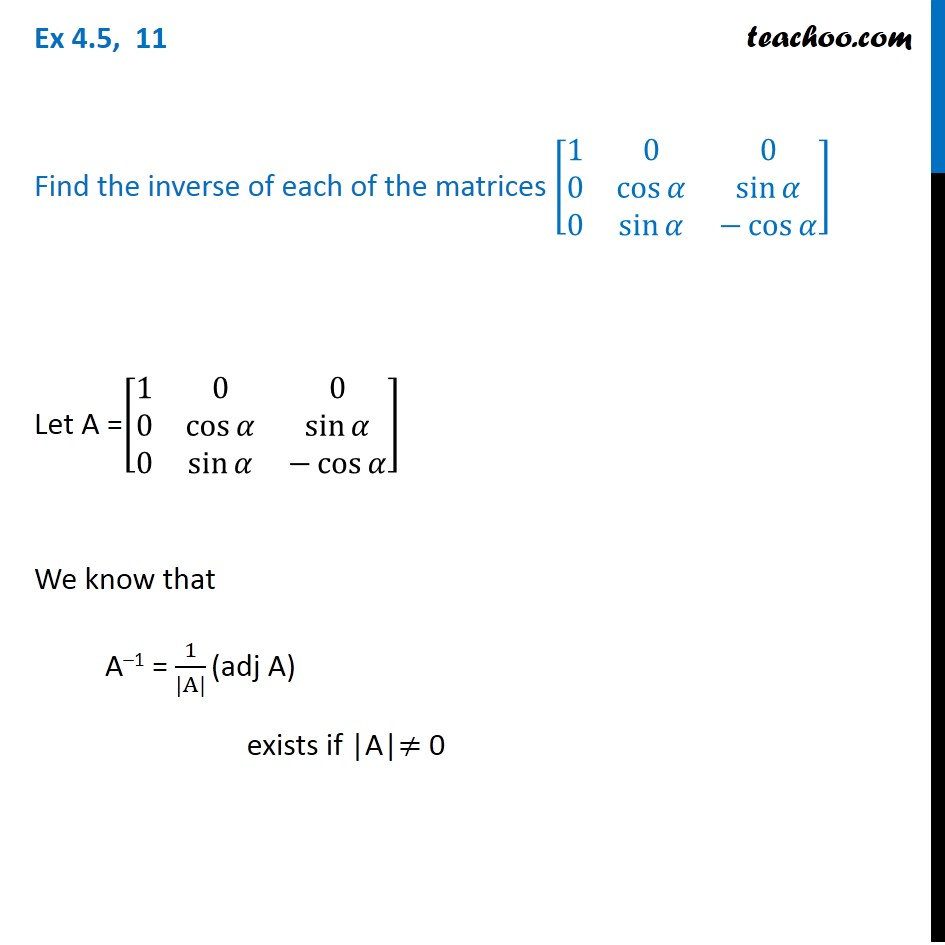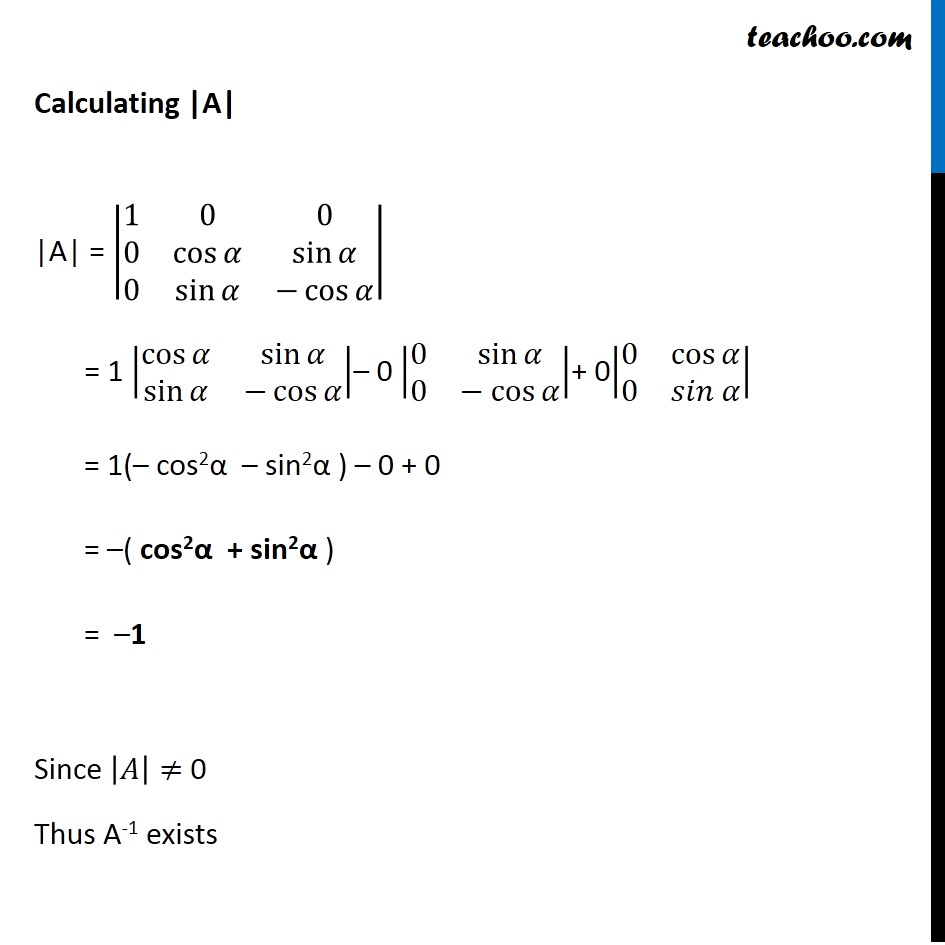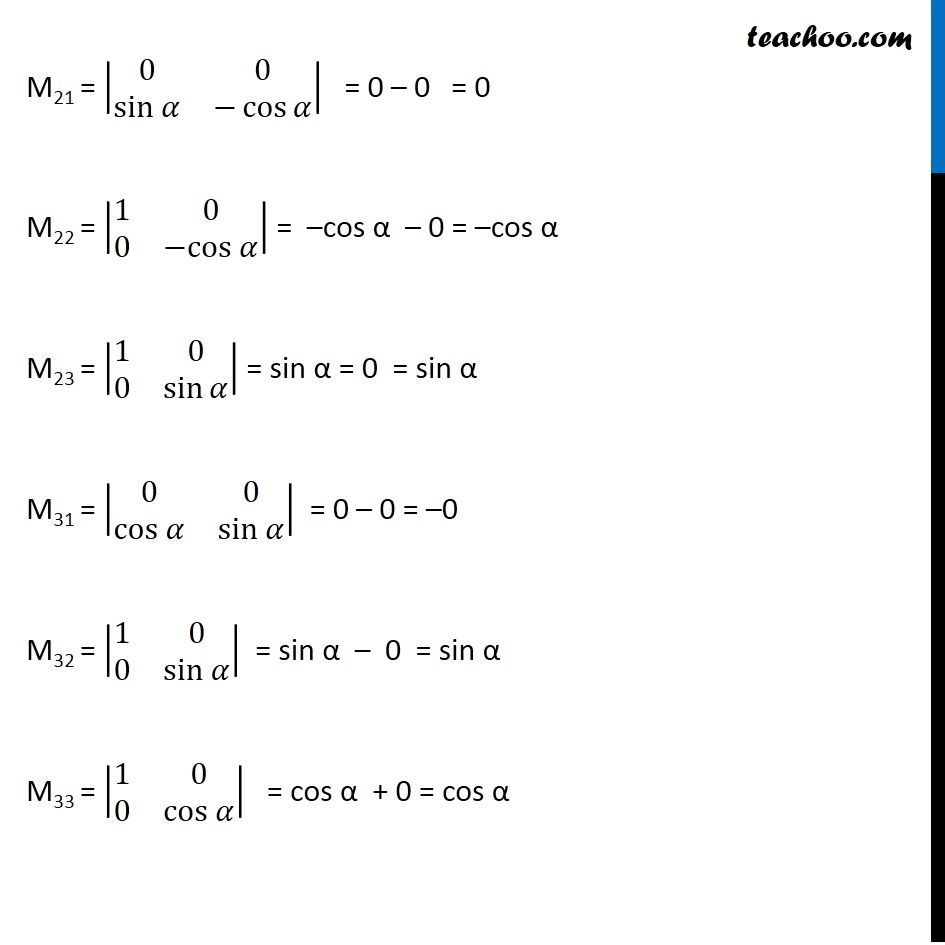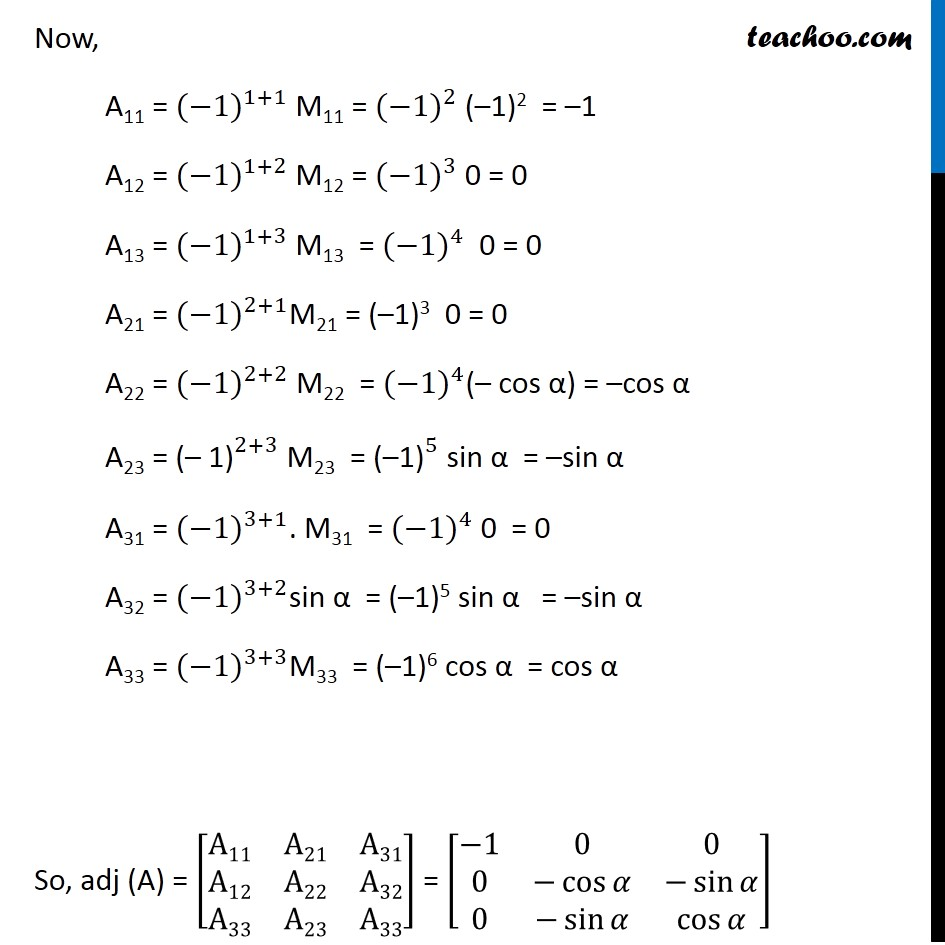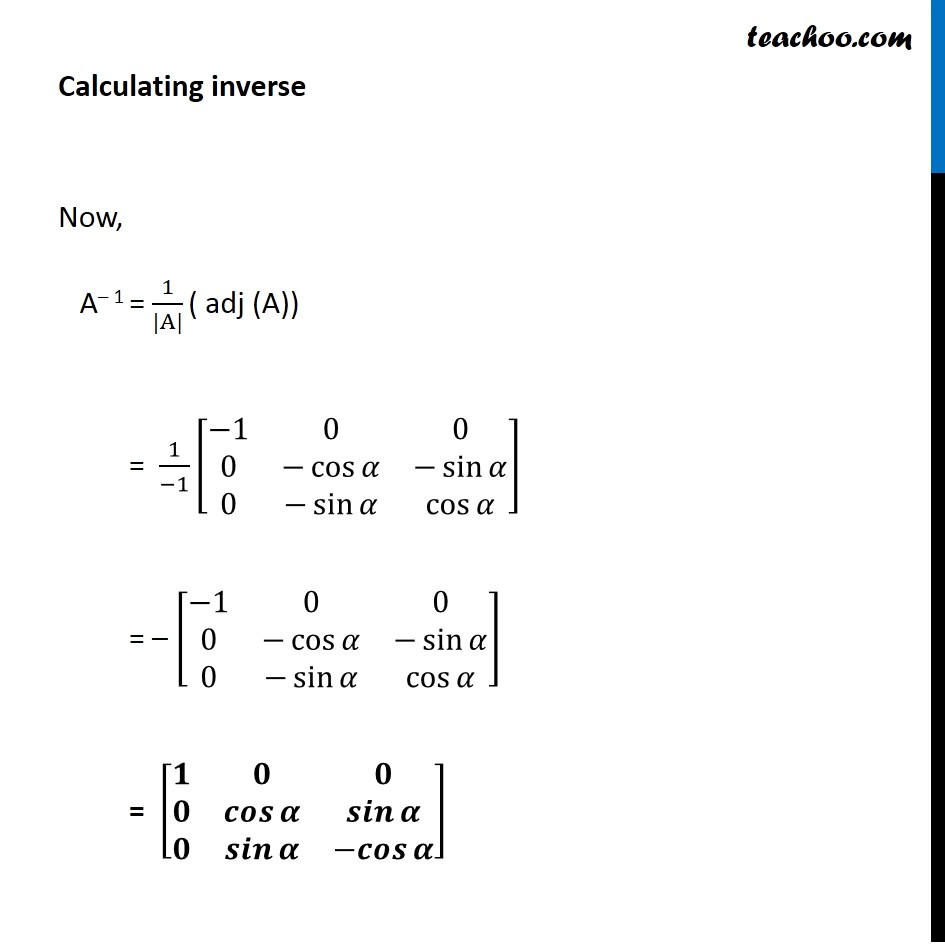Subscribe to our Youtube Channel - https://you.tube/teachoo

1. Chapter 4 Class 12 Determinants
2. Concept wise
3. Finding Inverse of a matrix

Transcript

Ex 4.5, 11 Find the inverse of each of the matrices [■8(1&0&0@0&cos⁡𝛼&sin⁡𝛼@0&sin⁡𝛼&−cos⁡𝛼 )] Let A =[■8(1&0&0@0&cos⁡𝛼&sin⁡𝛼@0&sin⁡𝛼&−cos⁡𝛼 )] We know that A–1 = 1/(|A|) (adj A) exists if |A|≠ 0 Calculating |A| |A| = |■8(1&0&0@0&cos⁡𝛼&sin⁡𝛼@0&sin⁡𝛼&−cos⁡𝛼 )| = 1 |■8(cos⁡𝛼&sin⁡𝛼@sin⁡𝛼&−cos⁡𝛼 )|– 0 |■8(0&sin⁡𝛼@0&〖− cos〗⁡𝛼 )|+ 0|■8(0&cos⁡𝛼@0&𝑠𝑖𝑛 𝛼)| = 1(– cos2α – sin2α ) – 0 + 0 = –( cos2α + sin2α ) = –1 Since |𝐴|≠ 0 Thus A-1 exists Calculating adj A adj (A) = [■8(A11&A21&A31@A12&A22&A32@A13&A23&A33)] A = [■8(1&0&0@0&cos⁡𝛼&sin⁡𝛼@0&sin⁡𝛼&−cos⁡𝛼 )] M11 = |■8(cos⁡"α" &sin⁡"α" @sin⁡"α" &−cos⁡"α" )| = –cos2α – sin2α = –(cos^2α 〖+ 𝑠𝑖𝑛〗^2α ) = –1 M12 = |■8(0&sin 𝛼@0&−cos 𝛼)| = 0 – 0 = 0 M13 = |■8(0&cos⁡𝛼@0&sin 𝛼)| = 0 – 0 = 0 M21 = |■8(0&0@sin 𝛼&−cos⁡𝛼 )| = 0 – 0 = 0 M22 = |■8(1&0@0&−cos 𝛼)| = –cos α – 0 = –cos α M23 = |■8(1&0@0&sin⁡𝛼 )| = sin α = 0 = sin α M31 = |■8(0&0@cos 𝛼&sin 𝛼)| = 0 – 0 = –0 M32 = |■8(1&0@0&sin 𝛼)| = sin α – 0 = sin α M33 = |■8(1&0@0&cos 𝛼)| = cos α + 0 = cos α Now, A11 = 〖(−1)〗^(1+1) M11 = 〖(−1)〗^2 (–1)2 = –1 A12 = 〖(−1)〗^(1+2) M12 = 〖(−1)〗^3 0 = 0 A13 = 〖(−1)〗^(1+3) M13 = 〖(−1)〗^4 0 = 0 A21 = 〖(−1)〗^(2+1)M21 = (–1)3 0 = 0 A22 = 〖(−1)〗^(2+2) M22 = 〖(−1)〗^4(– cos α) = –cos α A23 = 〖"(– 1)" 〗^(2+3) M23 = 〖"(–1)" 〗^5 sin α = –sin α A31 = 〖(−1)〗^(3+1). M31 = 〖(−1)〗^4 0 = 0 A32 = 〖(−1)〗^(3+2)sin α = (–1)5 sin α = –sin α A33 = 〖(−1)〗^(3+3)M33 = (–1)6 cos α = cos α So, adj (A) = [■8(A11&A21&A31@A12&A22&A32@A33&A23&A33)] = [■8(−1&0&0@0&−cos⁡𝛼&−sin⁡𝛼@0&−sin⁡𝛼&cos⁡𝛼 )] Calculating inverse Now, A– 1 = 1/(|A|) ( adj (A)) = 1/(−1) [■8(−1&0&0@0&−cos⁡𝛼&−sin⁡𝛼@0&−sin⁡𝛼&cos⁡𝛼 )] = – [■8(−1&0&0@0&−cos⁡𝛼&−sin⁡𝛼@0&−sin⁡𝛼&cos⁡𝛼 )] = [■8(𝟏&𝟎&𝟎@𝟎&𝒄𝒐𝒔⁡𝜶&𝒔𝒊𝒏⁡𝜶@𝟎&𝒔𝒊𝒏⁡𝜶&〖−𝒄𝒐𝒔〗⁡𝜶 )]

Finding Inverse of a matrix